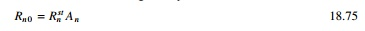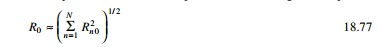Home | | Structural Dynamics and Earthquake Engineering | Spectrum analysis by modal response

# Spectrum analysis by modal response

In the case of design of structures, complete time history analysis is not necessary; it is enough to valuate the peak values of forces and deformations.

Spectrum analysis by modal response

In the case of design of structures, complete time history analysis is not necessary; it is enough to valuate the peak values of forces and deformations. A good estimate, although not accurate, of the peak response can be determined directly from response spectrum for the ground motion. These peak modal responses are combined using any one of the rules discussed below to get the value of the total response. This method is known as RSA.

For any response value such as force or deformation, the peak value Rn0 of the nth modal contribution is given bywhere An(Tn, Žün) is the ordinate of the pseudo-acceleration response spectrum corresponding to the natural vibration period Tn = 2ŽĆ/Žē and the damping ratio Žün. Rn0 is either positive or negative depending on whether Rnst is positive or negative.

1 Modal contribution rules

Knowing the peak value Rn0 of the nth mode contribution it is necessary to find the peak value R0 if the total response R(t). As we have seen in earlier chapters, the peak value Rn0 for each node occurs at different times and the combined peak response may attain its peak at a different time instance.

2 Absolute sum rule

If we assume that all peak values of modal responses occur at the same time, and ignore the algebraic sign for the peak value of the total response, we getThe above estimate is highly conservative and not popular in structural design applications.

The SRSS rule provides an estimate of peak value according to the equationThe algebraic sign of Rn0 does not affect the value of R0. This rule is applicable for structures where natural vibration frequencies are well separated. The SRSS rule was developed by Rosenblueth (1951).

4 CQC rule

The rule is applicable to wide range of structures in which natural frequencies of closely spaced such as those in unsymmetric buildings. According to the CQC rule (first developed by Rosenblueth and Elorduy)It is assumed that the damping ratio Žé is the same for all modes. Figure 18.27 shows the variation of correlation coefficient with respect to ╬▓ij. It is to be observed that the correlation coefficient Žüij is significant at ╬▓ij = 1.

In Žü matrix the leading diagonal terms are equal to 1. Hence Eq. 18.78 is rewritten asThe first summation of the right-hand side is identical to SRSS combination rule, whereas the next term may be positive or negative, depending the algebraic signs of Rn0. It is also seen from Fig. 18.27 that Žüij Ōēł 0 when Žēi, Žēj move farther apart. For well-separated vibration frequencies Žüij vanish. As a result the second term can be neglected and Eq. 18.83 reduces to the SRSS rule.5 Factors influencing the earthquake response

There are two parameters which influence the earthquake response: (1) fundamental natural vibration period T1 and (2) beam to column stiffness ratios based on the properties of the beam and column in the storey closest to the mid-height of the frame.where EIb and EIc are the flexural rigidities of beams and columns and Lb and Lc denote the lengths of the beams and columns. If ╬│ ŌåÆ Ōł× the beam imposes no restraint on joint rotations and the frame will bend as a flexure beam. If ╬╗ > Ōł× the structure will behave like a shear beam with double curvature bending in each column. An intermediate value of ╬│ represents the column and beam undergoing double curvature bending. This property ╬│ controls several properties of the structure when ╬│ is small and the vibration periods are more separated.

As the fundamental period increases with the velocity and displacement-sensitive regions of the structure, the higher mode response generally becomes an increasing percentage of the total response. For design accuracy more modes should be included in the analysis of buildings with longer periods than the buildings with shorter period. More modes should be included in the analysis of buildings with smaller ╬│. In some codal provisions, we have to include the number of modes such that 90% of the total mass is the participating mass.

Study Material, Lecturing Notes, Assignment, Reference, Wiki description explanation, brief detail
Civil : Structural dynamics of earthquake engineering : Spectrum analysis by modal response |